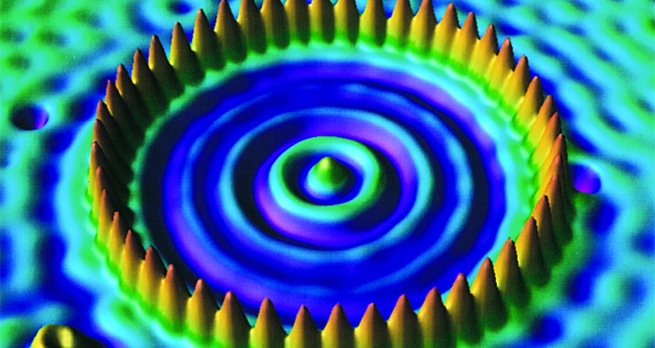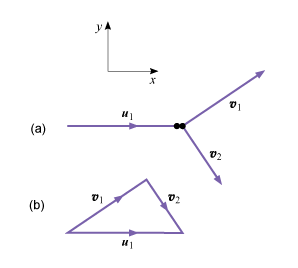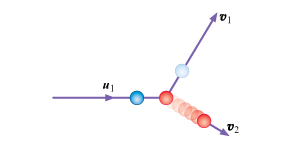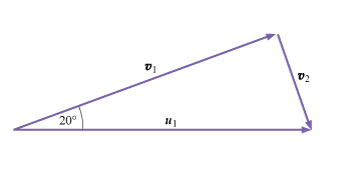Collisions and conservation laws

Start this free course now. Just create an account and sign in. Enrol and complete the course for a free statement of participation or digital badge if available.

Free course

# 4 Elastic collisions in two or three dimensions

The laws of conservation of momentum and energy that we used to analyse elastic collisions in one dimension are also used to analyse elastic collisions in two or three dimensions. We simply treat the motions in each dimension as independent, and apply conservation of momentum separately along each Cartesian coordinate axis. Kinetic energy conservation continues to provide one additional equation relating the squares of the particle speeds. Since we have been careful to use vector notation throughout, this extension to two or three dimensions is easily made.

Consider the elastic collision of two identical bodies of mass , one at rest and the other approaching with velocity . The particles are no longer confined to move in one dimension, so our -component equation (Equation 1), embodying conservation of momentum, becomes a full vector equation:

The law of conservation of energy (Equation 2) does not change, so

These can be simplified to:

Equation label: (10)

and

Equation label: (11)

These equations are most easily interpreted by a diagram. Figure 2 shows how the three vectors , and are related to one another. Equation 10 tells us that all three velocity vectors must lie in a single plane , and that they must form a closed triangle. Equation 11 tells us that the triangle must be a right-angled triangle, since its sides obey Pythagoras’ theorem. The implication of this is striking, it means that the angle between and must be .Figure 2 (a) Elastic collision between particles of equal mass, with one at rest; (b) the corresponding vector triangle.

Following the elastic collision of two identical particles, one of which is initially at rest, the final velocities of the two particles will be at right-angles.

This is a simplifying feature of equal-mass collisions in two or three dimensions, analogous to the simple result of the exchange of velocities, which we found in one dimension.

You may have noticed that this result does not tell us exactly where the bodies go after the collision. Any pair of final velocities which can be represented by Figure 2 will be equally satisfactory, and there are an infinite number of these. The reason for this is that we have said nothing about the shape or size of the bodies, or just how they collide. We usually need to have additional information of this kind if we are to determine unique final velocities in such cases. Figure 3 shows the outcome of a particular collision in which spherical bodies make contact at a specific point. The location of this point is the sort of additional information required to determine unique values for and .Figure 3 When ball strikes ball , the reaction forces at the contact point ensure that ball is propelled away along the line of centres, as in snooker.

## Activity 7

For the case illustrated in Figure 2 (two bodies of equal mass, one of which is initially at rest), if the moving body has an initial speed of , and is deflected through in the collision, find the magnitudes and directions of the velocities and .

We draw a vector triangle like the one shown in Figure 2bFigure 4 Vector triangle

We can now see that

and is at the given angle of to the -axis; has a magnitude

and must be at to the -axis so that the two angles add up to .

## Activity 8

In the same situation (Figure 2), if, instead of the outcome specified in Activity 7, the speed of the moving body is reduced from to by the collision, find the final velocities.

Using the triangle in Figure 2 Pythagoras’ theorem tells us that , so

so that .

Now is at an angle and at an angle to the -axis. You will observe that the two angles add up to , as they should.

When the masses of the two colliding particles are unequal the algebraic manipulations required to solve elastic collision problems become rather complicated, but no new physics is involved in the solution so we will not pursue such problems here.

S217_1